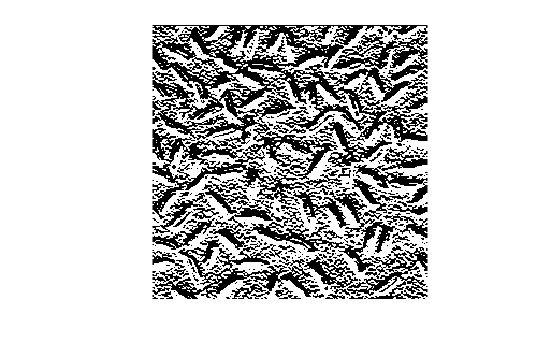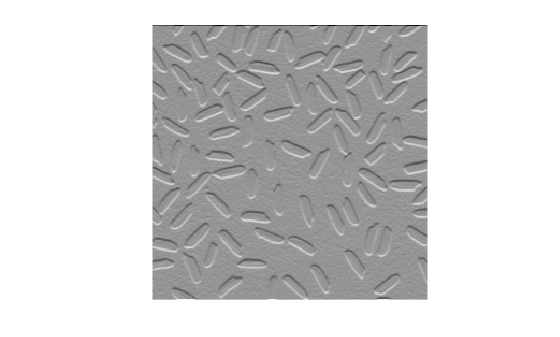mat2gray

Convert matrix to grayscale image

Description

I = mat2gray(A,[amin amax]) converts the matrix A to a grayscale image I that contains values in the range 0 (black) to 1 (white). amin and amax are the values in A that correspond to 0 and 1 in I. Values less than amin are clipped to 0, and values greater than amax are clipped to 1.

example

I = mat2gray(A) sets the values of amin and amax to the minimum and maximum values in A.

Examples

collapse all

Read an image and display it.

figure
imshow(I)Perform an operation that returns a numeric matrix. This operation looks for edges.

J = filter2(fspecial('sobel'),I);
min_matrix = min(J(:))
min_matrix = -779
max_matrix = max(J(:))
max_matrix = 560

Note that the matrix has data type double with values outside of the range [0,1], including negative values.

Display the result of the operation. Because the data range of the matrix is outside the default display range of imshow, every pixel with a positive value displays as white, and every pixel with a negative or zero value displays as black. It is challenging to see the edges of the grains of rice.

figure
imshow(J)Convert the matrix into an image. Display the maximum and minimum values of the image.

K = mat2gray(J);
min_image = min(K(:))
min_image = 0
max_image = max(K(:))
max_image = 1

Note that values are still data type double, but that all values are in the range [0, 1].

Display the result of the conversion. Pixels show a range of grayscale colors, which makes the location of the edges more apparent.

figure
imshow(K)Input Arguments

collapse all

Input image, specified as a numeric matrix.

Input black and white values, specified as a 2-element numeric vector.

• Values in input image A that are less than or equal to amin are mapped to the value 0 in the intensity image, I.

• Values in A that are greater than or equal to amax are mapped to the value 1 in I.

Output Arguments

collapse all

Output intensity image, returned as a numeric matrix with values in the range [0, 1].

Data Types: double